# Statistical signals (Bioinformatics)

## 1. Introduction

Detection of statistical signals in protein and DNA sequences is often a powerful method for uncovering biological significance and function. Examples of sequence features that can be identified through their statistical properties include protein motifs (see Article 28, Computational motif discovery, Volume 7), gene promoters, enhancers and suppressors (see Article 19, Promoter prediction, Volume 7 and Article 24, Exonic splicing enhancers and exonic splicing silencers, Volume 7), transcription binding sites, exon/intron splice junctions, regions of homology between sequences (see Article 39, IMPALA/RPS-BLAST/PSI-BLAST in protein sequence analysis, Volume 7), and protein docking sites. Each generally possesses some unique statistical property, or signal, that distinguishes it from other sequence segments. Signals may be interesting in their own right but are often combined in algorithms such as multiple alignments, homology detection, and gene finding (see Article 13, Prokaryotic gene identification in silico, Volume 7, Article 14, Eukaryotic gene finding, Volume 7, Article 20, Operon finding in bacteria, Volume 7, Article 21, Gene structure prediction in plant genomes, Volume 7, Article 25, Gene finding using multiple related species: a classification approach, Volume 7, and Article 27, Gene structure prediction by genomic sequence alignment, Volume 7).

## 2. Maximal aggregate scoring segments

Central to identification of statistically significant signals, either in individual sequences or when comparing a group of sequences, is the general scoring scheme (Karlin and Altschul, 1990). Given a score defined on individual letters of a sequence (e.g., the amino acid substitution matrices BLOSUM62 and PAM120, commonly used in sequence alignment; for recent developments, see Yu et al., 2003), the general problem is to determine the statistical significance of a sequence region exhibiting a high aggregate (additive) score. Let S be a sequence composed of letters from an alphabet A = {ai, a2, … , ar} that are independently randomly distributed with probabilities [pi, p2, … , pr}. For each letter in S, one can associate a score si (e.g., the score for a matching letter in another sequence). In general, we are interested in finding the segment of the sequence that has the maximum aggregate score. Two important conditions are applied. First, we require that at least one score be positive. Second, we require the expected score per letter,£ = Y, pisi , be negative. If E were positive, the maximal segment would tend to be the whole sequence. The maximal aggregate scoring segment Mn in a sequence of length occurs with probability given by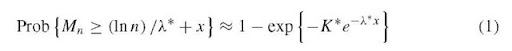where X* is the unique positive solution of J^’i=l pieX*Si = 1 , and K* is determined from a rapidly convergent series (see Karlin and Altschul, 1990). This approach can be extended to allow for neighbor dependencies (e.g., Markov models), as well as random and vector scores. To use the maximal scoring formulas, one chooses a significance level p (e.g., p = 0.001) and solves for x = x(p) so that a segment score exceeding (ln n)/X* + x(p) is significant at the p level. The homology search algorithm, BLAST applies the ideas of maximal aggregate score to find matching sequence blocks between query and database sequences (Altschul et al., 1997).

For example, consider the case of aligning two sequences where gaps are not allowed (Dembo et al., 1994). The first sequence is composed of letters ai with probability pi, and the second sequence of letters aj with probability p’j, so that the pairing of ai with aj occurs with probability pip’j. Assuming that the average pair score J2itj pipjsij is negative, then X* is the unique positive solution of pipj exp[X*sij} = 1. If the sequence lengths m and n grow at roughly similar rates, then equation (i) holds for the maximal scoring segmental alignment with n replaced by mn: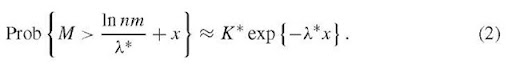In other words, the alignment of two sequences has an unusually high (statistically significant at the p = 0.01 level) score if M exceeds (ln nm)/X* + x0, with x0 determined so that K*exp{-X*x0} = 0.01. The number of “separate” high-scoring segments is close to a Poisson distribution with parameter K*exp{-X*x0}. This distribution can be used to estimate whether the number of high-scoring segments over a whole protein is unusually high. The theory readily generalizes to the case of multiple sequence alignments (Dembo et al., 1994).

The above results can be used in discriminating among possible scoring schemes. Suppose that [pi} are the overall letter frequencies in some random reference sequence. Now, let {qi} be desirable target frequencies that correspond to known representatives of some type of protein sequence that we wish to identify. Then, in a high-scoring segment, qi « pi exp[X*S;-} , and appropriate scores are naturally defined as si = ln(qi/pi), With these log ratio scores, the mean score for a sample letter is automatically negative. The PAM and BLOSUM matrices are examples of natural log odds ratio score matrices.

## 3. Significant segment pair alignments (SSPA)

Most measures of similarity between protein sequences are local. One way to score global similarity between two protein sequences A and B is as follows: determine all high-scoring sequence segment pairs (HSSPs) significant at the p =0.01 level. A consistent matching array is defined as a combination of HSSPs (optimizing overlaps) into a single “gapped” alignment. The significant segment pair alignment (SSPA) score for the sequence A and B is the maximal value with respect to all sets of consistently matching arrays, obtained by summing the segment scores. Normalization is then performed so that the SSPA score is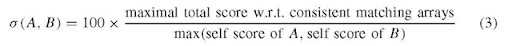or, alternatively, normalization can be done with respect to the minimum of the two self-scores. This normalization allows comparison of proteins of different sizes and quality. In either case, 0 < a(A,B) < 100. For sequence pairs with at least one significant SSPA match, additional matching segments are identified at a lower probability threshold (typically p = 0.50) and appended to the alignment. The secondary lower threshold helps to fill in gaps between significant HSSPs. The SSPA method can be incorporated into a multiple alignment algorithm that employs dynamic programming to accommodate insertions/deletions and variable-length unaligned segments (Brocchieri and Karlin, 1998).

## 4. Analysis of marker arrays: r-scan analysis

A frequent problem in sequence analysis is to determine the statistical significance of spacings between specific markers distributed throughout a sequence. For example, one might seek to determine whether direct repeats or dyads (a sequence fragment followed by its reverse complement) are significantly clumped (many neighbors with short spacings) or over-dispersed. The method of r-scans provides a rigorous framework for such determinations (reviewed in Reinert et al., 2000). Let the r-scan length be the distance between marker i and marker i + r, that is the cumulative length of r distances between markers. Extremal statistics for the {Rir)} can be determined from their limiting distributions. The distribution of a marker is determined by comparing the distribution of {R^} , calculated for a random sequence to those actually observed. Varying r permits detection of clustering or dispersion at different scales. Let the minimum and maximum r-scans be m* = mini Rir) and M* = max, R. The theoretical probabilities for a marker array of n points obey the asymptotic relations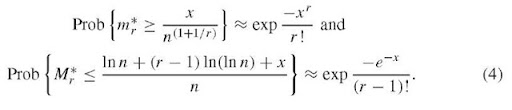These equations provide thresholds for whether minimum and maximum observed spacings deviate significantly from random expectation. For example, setting the probability on the left-hand side to a required significance (typically 0.0i) yields the formula exp(-xb/r.) = 0.01, which is solved for xb, and the threshold value b* = xb/n(l+l/r) is then determined. For a sequence of length L, observed r-scan lengths of m* < b*L define r-scan clusters. Similarly, significantly even spacing is indicated by m* > a*L, where ar is determined from equations (4) by setting the probability to 0.99. Analogous expressions can be obtained for the maximal spacing Mr*, and markers are considered to be distributed randomly if the r-scan lengths are in the ranges (a*L, b*L) and (A*L, B*L). r-scan statistics can also identify whether counts in sliding window plots are significant. For a window of size w , let N(t) be the number of markers in (0,t). The critical value r* that corresponds to a significance value p (e.g., p = 0.01) for the marker counts in the window can be calculated from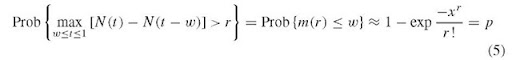where m (r) is the length of the minimum r-scan. Defining n by x = wni+i/r gives n(nw)r/r! + ln(i-p) = 0, which can be solved numerically to give r* for values of n, w, and p. This sliding window method correctly predicts the region of the lytic origin of replication for human cytomegalovirus (Masse et al., 1992).

## 5. Frequent words (oligonucleotides and peptides)

Another topic of interest is determination of sequence words that occur statistically more, or less, frequently than would be expected from random chance. For a sequence of length L, comprising letters drawn from an alphabet of size A, there is a natural word length s defined by the inequality As-i < L < As and a natural copy number r determined by (r – i)/r < (logL)/log As < r/(r + i). For sufficiently large L, the number of words of length s that occur more than r times is approximately Poisson distributed. This model, based on approximations to Poisson distributions concerned with generalized occupancy problems of balls-in-urns, works well in the case where the letter frequencies are roughly equal for the sequence (Karlin and Leung, 1991). In the case of a biased sequence, for example, an A + T rich genome such as Haemophilus influenzae, the method can be generalized to the case of words w that have separate frequencies pw. The word size s is as defined previously, but the copy number threshold, rw, for a particular word now satisfies pwL exp(-pwL)/rw. < i/L. In this formalism, the lower the expected frequency of the word, the lower the cutoff for it to be considered frequent. If the inequality is saturated, at most, one frequent word is expected in a random sequence of the same length. For independent letters, pw = f if 2 … fs, where fi is the frequency of occurrence of the i th letter in the sequence. For a Markov model, with transition probabilities fi,j between the i th and j th letters, pw = f if lj2 … fs-l,s. For the H. influenzae genome, L & i.8 million bp, which, with A = 4 for a nucleotide alphabet, gives a natural frequent word length of s = 9 bp. Applying the copy number threshold reveals that, amongst others, the sequence AAGTGCGGT and its inverted complement qualify as frequent words. These 9-mers can be recognized as the DNA-uptake signal sequences of H. influenzae, which are highly enriched in the genome of this bacterium and which facilitate the binding and uptake of H. influenzae’s own DNA or DNA from closely related species. In Escherichia coli, REP elements and Chi sites qualify as frequent words, along with pentapeptides corresponding to the ATP-binding domain. For Synechocystis, the palindrome GGCGATCGCC is highly frequent and significantly evenly distributed. In eukaryotes, amino acid frequent words include words characteristic of zinc fingers (CGKAF, CEECG), chymotrypsin proteases (LTAAH, GDSGGP), serine/threonine and tyrosine kinases (ADFGL, FGQGT), and homeobox proteins (FQNRR, HFNRY).

## 6. Repeat structures

The patterns of significantly long repeats, frequency, length, and genomic environment may provide insights on evolution of genomes. All large repeats of the H. influenzae genome can be determined, allowing for error blocks (Leung et al., 1991). There are 150 occurrences in 51 repeat families; all involve at least 75% identities:

 Sizes (bp) 25-29 30-39 40-59 60-99 100-139 140-179 180-399 400-699 700-1699 =3000 Occurrences 14 18 17 14 19 17 19 19 10 3

Spacings between repeats:

 tandem 2bp-1 kb 1-10kb 10-200kb =200 kb Number of matches 18 8 7 28 33

Number of occurrences in distinct repeat families:

 Family size 2 3 4 5 6 13 14 Count of families 38 5 3 2 1 1 1

Some atypical examples of direct repeats in H. influenzae include the following: (1) A ~3.2-kb direct repeat (97% identity). The first occurrence at about 1371-1380 kb in the published sequence contains two major insertions (0.9 and 4.2 kb long). The second uninterrupted occurrence is at 1416-1420 kb. (2) A 600-bp tandem-perfect direct repeat (one base insertion) spans the 3′ part of gene guaB and the 5′ part of gene guaA. (3) Three copies of ~500bp with 97% identical sequences, all intergenic, around positions 173, 723, and 1344 kb.